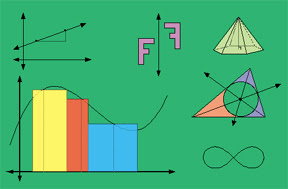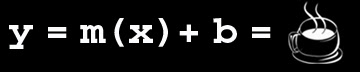Saturday, December 23, 2006

Write This Expression In Point-Intercept FormThis morning I got up and thanks to some quick thinking on my part, using the point-intercept form of the lines represented by my kitchen table legs and table-top, I quickly calculated the slope of the surface options I had to set down my full cup of coffee.

I really needed the coffee this morning, and did not want to risk losing it to the floor.

With my Blackberry in my free hand, and using only my right thumb while cradling the device in my palm, I quickly worked through the problem:

y = m(x) + b, where m is the slope and b is the y-intercept. Slope-intercept is the form used most often as the simplified equation of a line. See also Point-slope, standard form, two intercept, vertical line and horizontal line definitions.Let’s see… the table is about 40 inches high, so the y intercept would be 40, while the x-intercept would also be 40 (where the x line is the table leg, and y was the table surface). Therefore, I can assume one of the the points on the graph would be (40,40). So I can write the expression in this way:

40=40m+40

Ok, so then I subtract 40 from the right hand of the equation, and also from the left, leaving:

0=40m

Almost there. So, anything multiplied by 0 equals 0, so that means that m must equal 0. And finally, if m, representing the slope, was 0, then the surface of the table had no slope and my coffee cup was safe. If, however, I place my coffee cup on the surface of the table leg, with a slope of “undefined” I would have problems.

Awesome! I have finally figured out how to make algebra a part of my daily life.

I knew in high school, even middle school, that I would not be an architect, scientist, astronaut, pilot, financial planner, or even an algebra teacher. I fought all the way through school asking why I needed to know this stuff. The answers, and yes, there are at least 3x of them, are now evident to me:

1. You must learn algebra to prove to the right-brain academicians that you are not just a feel-good, mushy, artsy type. Or a jock.

2. You must learn algebra because it teaches you critical thinking patterns that helps you in all areas of life (this one is expressly for my daughter Ks benefit. She reads my blog, and I don’t want her throwing this stuff back at me and asking me irritating questions like “so why do I have to learn algebra anyway?”).

3. You must learn algebra because your thirteen year old daughter will ask for help studying for her final exam right before Christmas break.